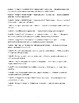# 36 Puzzle Calculus Package,No Calc AB, 30 Mathacrostics + 6 Other PuzzlesSubject
Resource Type
File Type

PDF

(3 MB|84 pages)
Product Description

The Three Pre Calculus and Three Post Calculus Puzzles

This is a six puzzle set of pre- and post- calculus course puzzles that are different from the “mathacrostics” puzzles. Three of the puzzles might best be given before the study of Calculus begins, 3 would best be given after the study of Calculus is completed. The six puzzles are: First, a Pre Calculus Mathematician Search wherein the puzzle-doer will search in a "word search" type puzzle for many of the mathematicians, ancient and later, who made contributions to the foundation of what was needed to "build" calculus. Upon conclusion of the search when the uncrossed out letters are arranged in order an apt message can be found. Second, a Calculus History Essay wherein the puzzle-doer will have to work with some of the exponents that will come up throughout the year when differentiating and, particularly so, when integrating to complete the essay/puzzle. Upon conclusion of the review/essay there's a fill in the blanks "secret message" that gives an apt definition. Third, a Pre Calculus Calculus Beginnings and a Little Math Crossword wherein the puzzle-doer will work on a crossword puzzle involving Calculus and Math. The 17 Calculus, Calculus mathematicians, and Math facts clues are all in bold-faced print. Fourth, a Post Calculus Mathematician Search wherein the puzzle-doer will search in a "word search" type puzzle for many of the mathematicians who made contributions that have made Calculus what it is today. Upon conclusion of the search when the uncrossed out letters are arranged in order an apt message can be found. Fifth, a Calculus Formulas Matching Puzzle wherein the puzzle-doer will have to pair up entries in a left hand column that has formulas and expressions from calculus with a right hand column that is composed of what the formulas will get. Upon conclusion of the review/essay there's a fill in the blanks "secret message" that gives an apt definition. And sixth, a Post Calculus Some Calculus, Some Mathematicians and More Math Crossword wherein the puzzle-doer will work on a crossword puzzle involving Calculus and Math. It is intended to be done AFTER the course in Calculus is completed. The 19 Calculus, Calculus mathematicians, and Math facts clues are all in bold-faced print.

The No Calculator, AP AB Calculus 30 Mathacrostics Puzzles

These individual puzzles, and the Calculus Mathacrostic Puzzle book they will make up are intended:

1. to be a “last second” no-calculator Advanced Placement preparation review of a, perhaps,

forgotten topic in first quarter Calculus;

2. to be a more pleasant additional assignment (with mostly nice and semi-nice answers) on

topics that the teacher feels a class needs more work on;

3. to be given to an individual student who the teacher has noticed needs more work in a

certain area (which is why complete solutions of all problems are provided with each

puzzle);

4. to be used by the teacher as a source for their own test/quiz questions (which is why

complete solutions of all problems are provided with each puzzle);

5. to be used as a day-before-vacation-classroom-or-take-home-assignment (which is why

complete solutions of all problems are provided with each puzzle);

6. to be used as an assignment given when “bad weather and book bag” assignments are

anticipated (which is why complete solutions of all problems are provided with each

puzzle).

I will definitely admit that you, the teacher, and probably many of your better students, will be able to solve several of the puzzle parts by just matching answer choices with puzzle questions and not having to work the entire problem. But most calculus students are pretty concerned about actually understanding the concepts involved and will actually do the 15 to 20 problems involved in each puzzle – particularly if you insist that their work be shown.

I’ve tried to run the gamut of problem difficulty – from easy to somewhat messy. I’ve also tried to pick topics that are “true Calculus” concepts as opposed to pre-Calculus topics. “No-calculator” problems on the AP Calculus tests generally work out nicely – but having to deal with some messy arithmetic for a little practice will certainly do no harm. In this age of calculator and phone dependency it is, for the most part, direly needed.

Total Pages
84 pages
Included
Teaching Duration
1 Year
Report this Resource to TpT
Reported resources will be reviewed by our team. Report this resource to let us know if this resource violates TpT’s content guidelines.